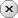### 用户注册### 用户登录### 发表随想lb0123    -  云代码空间

—— 自己 不努力每人会帮你

# python学习笔记

2020-01-28|50阅||

## 摘要:运算符与表达式

#算术运算：+ -  * / % ** // num1 = 2 num2 = 5 print('num1+num2=',num1+num2) print('num1-num2=',num1-num2) print('num1*num2=',num1*num2) print('num1/num2=',num1/num2) print('num1%um2=',num1%num2) print('num1**num2=',num1**num2) print('num1//num2=',num1//num2)
#if语句 if 1:  print('if 语句成功标志')
#if else 语句 if 0:  print('表达式为真') else:  print('表达式为假')
#输入读取 #flag=int(input('请输入0或1：')) flag = 0 if flag:  print('输入的值为真') else:     print('输入的值为假')     #and运算符 a=1 b=2 if a and b:     print("a，b都为真") else:     print(" a,b至少一个为假")
#or运算符 a=1 b=2 if a and b:     print("a，b至少一个为真") else:     print(" a,b都为假")
#not运算符 a=1 if not a:     print("a为假") else:     print("a为真")

阅读表达式：
1.表达式的功能
2.阅读表达式的值
算数运算符与算数运算表达式：
1.算数运算符：
+  -  *  /  %    **    //
加 减 乘 除 求余  求幂  取整
功能：进行符号对应算数符号算数运算，不会修改变量的值
值  ：相应算数运算的结果
例如：
num1 = 2
num2 = 5
print(num1+num2)
print(num1-num2)
print(num1*num2)
print(num1/num2)
print(num1%num2)
print(num1**num2)
print(num1//num2)
结果：
num1+num2= 7
num1-num2= -3
num1*num2= 10
num1/num2= 0.4
num1%um2= 2
num1**num2= 32
num1//num2= 0

2.赋值运算符和赋值运算表达式：
赋值运算符：   =
赋值运算表达式：
格式：变量 = 表达式
功能：计算右侧表达式的值并赋值给=左侧的变量
值 ： 赋值结束后变量的值
3.复合运算符：
+=  -=  *=  /=  %=  **=  //=
a +=b  ->  a=a+b
a -=b  ->  a=a-b
a *=b  ->  a=a*b
a /=b  ->  a=a/b
a %=b  ->  a=a%b
a **=b  ->  a=a**b
a //=b  ->  a=a//b
4.位运算符：
&  按位与运算符：参与运算的两个值，如果两个相应位都为1，这该位结果为1，否则为0 注意：需要用进制进行判断
例如： 7 & 5  换成二进制 111 & 101
111
101
------
101       换成十进制为5 所以7 & 5=5
|  按位或运算符：参与运算的两个值有一个为1，结果为1，否则结果为0
例如： 5|7=7
^  按位异或运算符：参与运算的两个值相同时结果为1，否则为0
例如： 5^7=5
~  按位取反运算符：对数据的二进制位进行取反操作，即把1变为0，把0变为1
例如： ~5
<< 左移运算符：  运算数的各二进制全部左移若干位，由<<右侧的数字决定移动的位数
例如： 0000000000000010十进制中的 2
2<<2=8   ->0000000000000010 << 0000000000001000
>> 右移运算符：运算数的各二进制全部右移若干位，由<<右侧的数字决定移动的位数
5.关系运算符与关系运算表达式：
1、if 语句表达式：
使用格式：if~表达式：
~~~~语句   （~表示空格）
if 语句的逻辑：
当程序运行到 if 语句时，首先进行计算表达式的值是否为真，若为真则执行语句
若为假则跳过语句
表达式为假的情况：0  0.0  ''（空字符串） None  False
表达式为真的情况：除了上述情况外都为真
2、if else 语句
格式：
if 表达式：
语句1
else：
语句2
逻辑：当程序运行到 if else语句时，判断表达式的值，若为真执行语句1，若为假执行语句2

关系运算符与关系运算表达式：
关系运算符分类： ==     !=       >     <      >=         <=
等于  不等于    大于  小于  大于等于  小于等于
格式：表达式1 关系运算符 表达式2
功能：计算表达式1和表达式2的值
值  ：如果关系成立，则整个关系运算表达式的值为真
若关系不成立，则整个关系运算表达式的值为假
逻辑运算符：逻辑与、逻辑或、逻辑非
逻辑与：
格式：表达式1 and 表达式2
功能：首先计算表达式1的值，若为真则计算表达式2的值
若表达式1的值为真，表达式2的值为真，则为真
若表达式1的值为真，表达式2的值为假，则为假
若表达式1的值为假，表达式2的值为真，则为假
若表达式1的值为假，表达式2的值为假，则为假
例如：a=1
b=2
if a and b:
print("a，b都为真")
else：
print(" a,b至少一个为假")
逻辑或：
格式：表达式1 or 表达式2
功能：首先计算表达式1的值，若为真则不再计算表达式2的值，直接判断为真
若表达式1的值为假，表达式2的值为假，则为假
若表达式1的值为假，表达式2的值为真，则为真
若表达式1的值为真，表达式2的值为假，则为真
若表达式1的值为真，表达式2的值为真，则为真
例如：a=1
b=2
if a or b:
print("a，b至少一个为为真")
else：
print(" a,b都为假")
逻辑非：
格式：not 表达式
值  ：如果表达式的值为真则逻辑非运算的值为假
如果表达式的值为假则逻辑非运算的值为真
总结：颠倒黑白
例如：
a=1
if not a：
print("a为假")
else:
print("a为真")
成员运算符：
格式：x in seq    x not in seq
in：如果在指定的序列中，则返回真
not in：如果不在序列中，则返回假
身份运算符：
格式：obj1 is obj2    obj1 is not obj2
is 判断两个标识符身份表示同一个对象，引用相同返回为真，否则为假
is not 判断两个标识符引用是否为不同对象，不同返回真，否则返回假

运算符的优先级：
**    指数
~ + - 按位取反和医院加减号即正负号
* / % //
+ -   加减号法
<< >>
&
| ^
< > <= >=
== !=
= *= /= %= //= += -= **=
is     is not
in     not in

发表评论

• 昵称： lb0123
• 等级： 中级程序员
• 积分： 257
• 代码： 7 个
• 文章： 8 篇
• 随想： 0 条
• 访问： 0 次
• 关注